# Difference between revisions of "Regularity criteria"

for summation methods

Conditions for the regularity of summation methods.

For a matrix summation method defined by a transformation of a sequence into a sequence by means of a matrix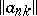,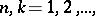the conditions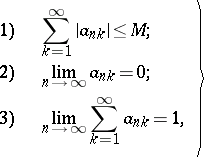(1)

are necessary and sufficient for regularity. For the matrix summation method defined by a transformation of a series into a sequence by means of a matrix,necessary and sufficient conditions for regularity are as follows: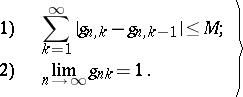(2)

The conditions (1) were originally established by O. Toeplitz  for triangular summation methods, and were then extended by H. Steinhaus  to arbitrary matrix summation methods. In connection with this, a matrix satisfying conditions (1) is sometimes called a Toeplitz matrix or a-matrix.

For a semi-continuous summation method, defined by a transformation of a sequence into a function by means of a semi-continuous matrix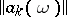or a transformation of a series into a function by means of a semi-continuous matrix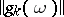, there are regularity criteria analogous to conditions (1) and (2), respectively.

A regular matrix summation method is completely regular if all entries of the transformation matrix are non-negative. This condition is in general not necessary for complete regularity.

How to Cite This Entry:
Regularity criteria. Encyclopedia of Mathematics. URL: http://encyclopediaofmath.org/index.php?title=Regularity_criteria&oldid=48488
This article was adapted from an original article by I.I. Volkov (originator), which appeared in Encyclopedia of Mathematics - ISBN 1402006098. See original article